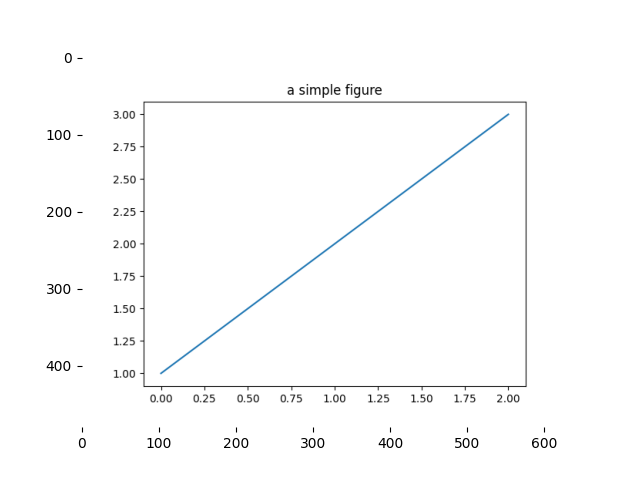# Agg Buffer To Array¶

Convert a rendered figure to its image (NumPy array) representation.import matplotlib.pyplot as plt
import numpy as np
from matplotlib.figure import Figure
from matplotlib.backends.backend_agg import FigureCanvas

# Create a figure that pyplot does not know about.
fig = Figure()
# attach a non-interactive Agg canvas to the figure
# (as a side-effect of the __init__)
canvas = FigureCanvas(fig)
ax = fig.subplots()
ax.plot([1, 2, 3])
ax.set_title('a simple figure')
# Force a draw so we can grab the pixel buffer
canvas.draw()
# grab the pixel buffer and dump it into a numpy array
X = np.array(canvas.renderer.buffer_rgba())

# now display the array X as an Axes in a new figure
fig2 = plt.figure()